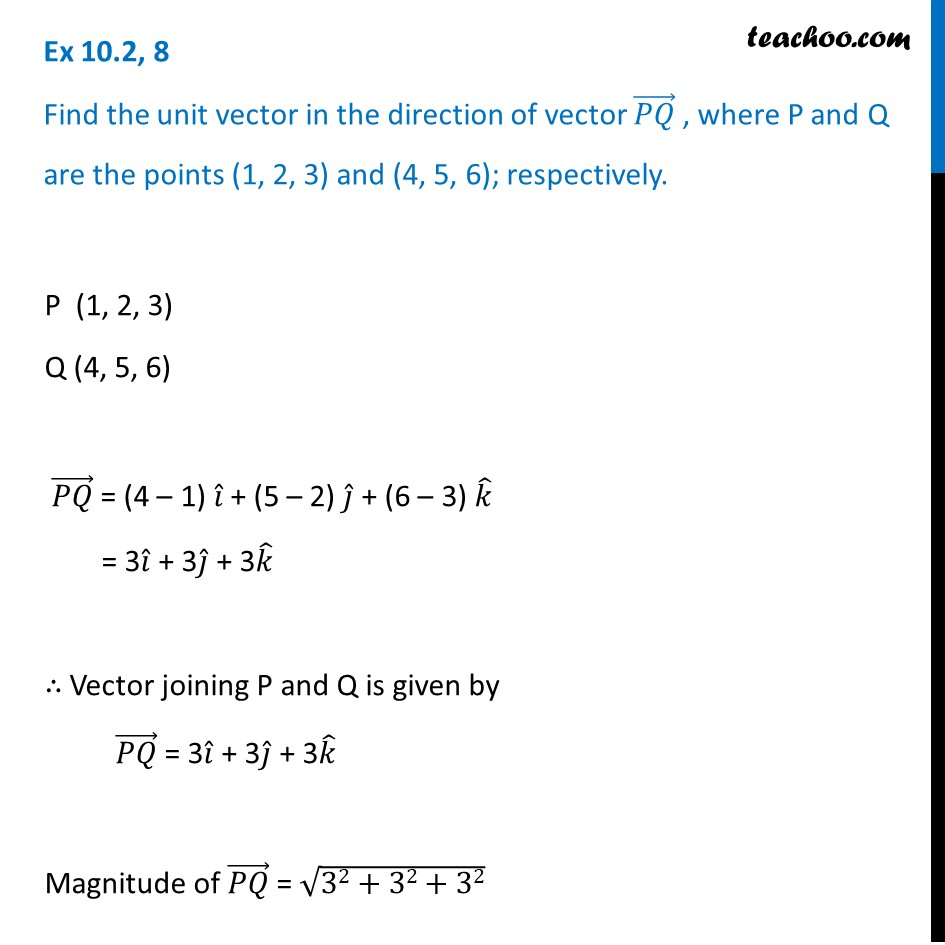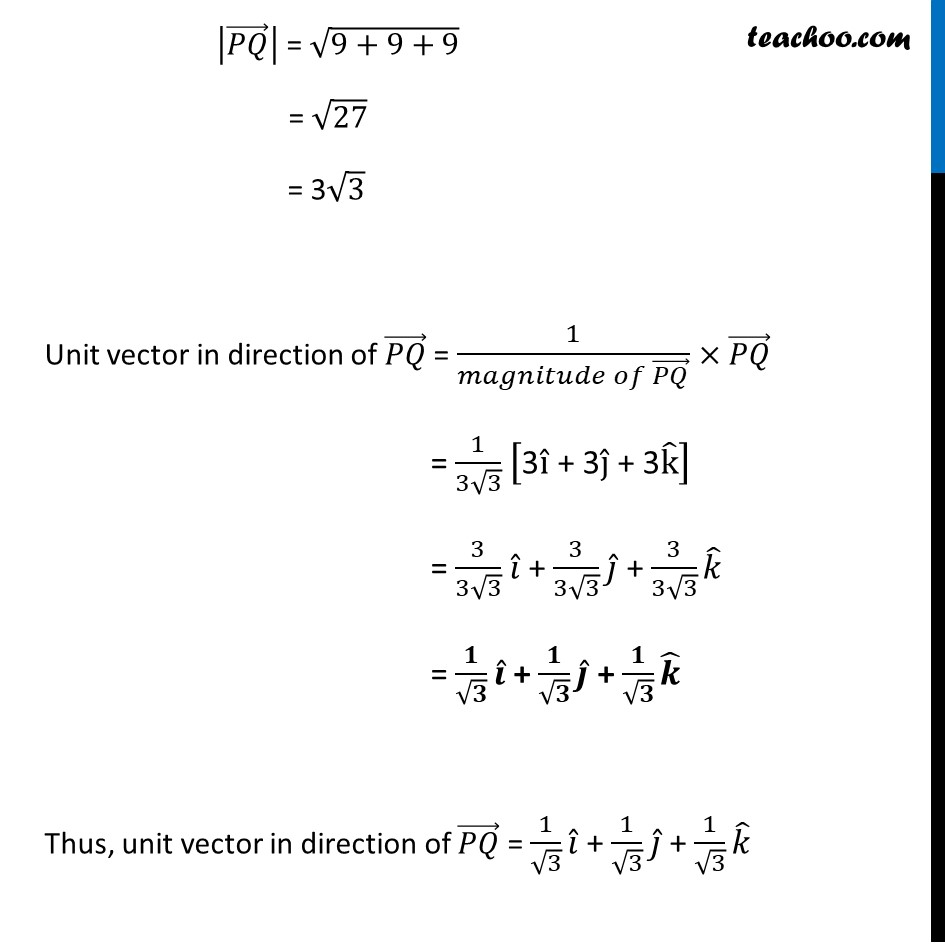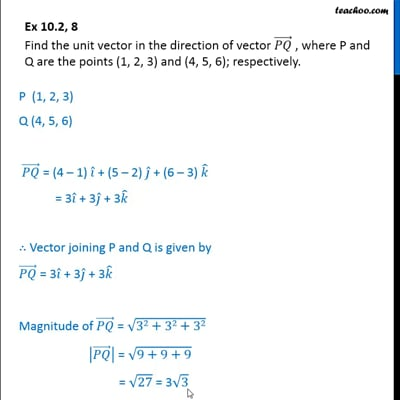Ex 10.2

Chapter 10 Class 12 Vector Algebra
Serial order wiseThis video is only available for Teachoo black users

Solve all your doubts with Teachoo Black (new monthly pack available now!)

### Transcript

Ex 10.2, 8 Find the unit vector in the direction of vector (𝑃𝑄) ⃗ , where P and Q are the points (1, 2, 3) and (4, 5, 6); respectively.P (1, 2, 3) Q (4, 5, 6) (𝑃𝑄) ⃗ = (4 – 1) 𝑖 ̂ + (5 – 2) 𝑗 ̂ + (6 – 3) 𝑘 ̂ = 3𝑖 ̂ + 3𝑗 ̂ + 3𝑘 ̂ ∴ Vector joining P and Q is given by (𝑃𝑄) ⃗ = 3𝑖 ̂ + 3𝑗 ̂ + 3𝑘 ̂ Magnitude of (𝑃𝑄) ⃗ = √(32+32+32) |(𝑃𝑄) ⃗ | = √(9+9+9) = √27 = 3√3 Unit vector in direction of (𝑃𝑄) ⃗ = 1/(𝑚𝑎𝑔𝑛𝑖𝑡𝑢𝑑𝑒 𝑜𝑓 (𝑃𝑄) ⃗ ) ×(𝑃𝑄) ⃗ = 1/(3√3) ["3" i ̂" + 3" j ̂" + 3" k ̂ ] = 3/(3√3) 𝑖 ̂ + 3/(3√3) 𝑗 ̂ + 3/(3√3) 𝑘 ̂ = 𝟏/√𝟑 𝒊 ̂ + 𝟏/√𝟑 𝒋 ̂ + 𝟏/√𝟑 𝒌 ̂ Thus, unit vector in direction of (𝑃𝑄) ⃗ = 1/√3 𝑖 ̂ + 1/√3 𝑗 ̂ + 1/√3 𝑘 ̂